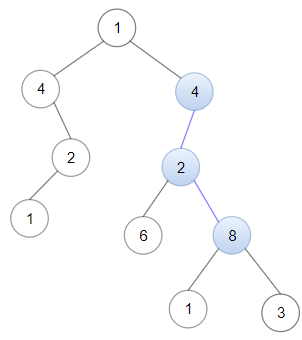Formatted question description: https://leetcode.ca/all/1367.html

# 1367. Linked List in Binary Tree (Medium)

Given a binary tree root and a linked list with head as the first node.

Return True if all the elements in the linked list starting from the head correspond to some downward path connected in the binary tree otherwise return False.

In this context downward path means a path that starts at some node and goes downwards.

Example 1:Input: head = [4,2,8], root = [1,4,4,null,2,2,null,1,null,6,8,null,null,null,null,1,3]
Output: true
Explanation: Nodes in blue form a subpath in the binary Tree.


Example 2:Input: head = [1,4,2,6], root = [1,4,4,null,2,2,null,1,null,6,8,null,null,null,null,1,3]
Output: true


Example 3:

Input: head = [1,4,2,6,8], root = [1,4,4,null,2,2,null,1,null,6,8,null,null,null,null,1,3]
Output: false
Explanation: There is no path in the binary tree that contains all the elements of the linked list from head.


Constraints:

• 1 <= node.val <= 100 for each node in the linked list and binary tree.
• The given linked list will contain between 1 and 100 nodes.
• The given binary tree will contain between 1 and 2500 nodes.

Related Topics:

## Solution 1.

// OJ: https://leetcode.com/problems/linked-list-in-binary-tree/

// Time: O(N * min(L, H))
// Space: O(H)
class Solution {
bool dfs(ListNode *head, TreeNode *root) {
if (!root) return false;
}
public:
bool isSubPath(ListNode* head, TreeNode* root) {
if (!root) return false;
}
};


Java

/**
* public class ListNode {
*     int val;
*     ListNode next;
*     ListNode(int x) { val = x; }
* }
*/
/**
* Definition for a binary tree node.
* public class TreeNode {
*     int val;
*     TreeNode left;
*     TreeNode right;
*     TreeNode(int x) { val = x; }
* }
*/
class Solution {
public boolean isSubPath(ListNode head, TreeNode root) {
searchQueue.offer(root);
while (!searchQueue.isEmpty()) {
TreeNode node = searchQueue.poll();
listQueue.offer(node);
TreeNode left = node.left, right = node.right;
if (left != null)
searchQueue.offer(left);
if (right != null)
searchQueue.offer(right);
}
while (temp != null && !listQueue.isEmpty()) {
int nextVal = temp.val;
int size = listQueue.size();
for (int i = 0; i < size; i++) {
TreeNode node = listQueue.poll();
TreeNode left = node.left, right = node.right;
if (left != null && left.val == nextVal)
listQueue.offer(left);
if (right != null && right.val == nextVal)
listQueue.offer(right);
}
temp = temp.next;
}
return !listQueue.isEmpty();
}
}